FAQ – DACs

What does a DAC do logically?

A Digital-to-Analog Converter (DAC) is a device that converts digital data to an analog signal. It takes the binary input bits, weights and adds these together. Electrically SHF DACs do much more because of the on-chip 3R regeneration (re-timing, re-shaping & re-amplification) but logically this is the only operation a DAC does.

To have it less abstract, let’s assume a 3 Bit DAC in symmetric configuration (i.e. with equal amplitudes steps between the output levels) and for the sake of getting an overview, let’s normalize the voltage contributions. The output amplitude can be realized by adding the individual levels (basically it is just like counting binary). The table below shows a typical 3-Bit DAC scheme:

 D2 D1 D0 Norm. Voltage Output of a DAC 1 1 1 → 4+2+1 = 7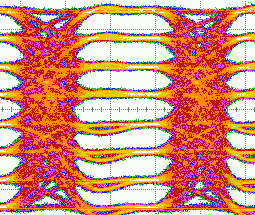1 1 0 → 4+2+0 = 6 1 0 1 → 4+0+1 = 5 1 0 0 → 4+0+0 = 4 0 1 1 → 0+2+1 = 3 0 1 0 → 0+2+0 = 2 0 0 1 → 0+0+1 = 1 0 0 0 → 0+0+0 = 0

This scheme holds true for the 3-Bit DAC as above as well as for the 6-Bit DAC. However, for a 6-Bit DAC one has 26=64 instead of the 23=8 output levels shown above. There is no other difference between a 6 and a 3-Bit DAC. The more bits are used, the more levels the output has (with means greater resolution).

Can I use the SHF DAC with less input bits?

Yes, not all data inputs of the SHF DAC must be used. For example, in case a PAM-4 signal shall be generated only two data input signals must be applied to the DAC. All other inputs can be left floating.

Even operating the DAC as a binary D-type Flip Flop by using one input bit only is possible.

For such cases it is recommended to use the most significant bits of the DAC as these provide the biggest contribution to the output signal amplitude. In other words, the output amplitude is higher if the more significant bits are used and therefore the signal-to-noise ratio will be improved.

What voltage levels do I get from the DAC? Can I adjust the individual eye openings?

As an example, for a 3-Bit DAC, the relation between output voltage Uout and the input data D1, D2 and D3 is calculated as follows:

Uout=D0 · a0 + D1 · a1 + D2 · a2 .

In the data sheet of the SHF DACs you will find a table showing the maximum contribution of each bit similar as below:

 Input D2 Input D1 Input Do Output Amplitude Output Amplitude (example SHF 615 A) on a0max 345 mV on a1max ≈ 2·a0max 690 mV on a2max ≈ 2·a1max ≈ 4·a0max 1380 mV on on on a0max + a1max + a2max 2415 mv

The amplitude contribution of each individual input Bit is adjustable by the appropriate slider in the GUI. The minimum voltage contribution anmin is half of the maximum anmax. As the DACs are calibrated, the voltage contribution in Volts is shown in the GUI.

To understand what implications this has on a PAM signal, lets assume the DAC is used in PMA-4 / 2-Bit Mode, i.e. the voltage contribution can be calculated as follows:

Uout=D1 · a1 + D2 · a2 .

For the PAM-4 signal this has the following implications:

• The output amplitude of a 3-Bit DAC in 2-Bit mode (one bit unused) is not as high as with all three bits used.
• If a1 & a2 are reduced by the same factor the overall amplitude gets reduced. By setting all contributions to the minimum the output power is reduced by 6 dB.
• If a1 is reduced (and/or a2 is raised) the inner of the three individual eyes gets bigger. In other words, the symmetry is changed. Please note, the symmetry cannot be changed in case all input bits are used.
• Adjusting all eyes individually (e.g. making the lower eye smaller, while enlarging the upper eyes) is not possible. Such unequal PAM signals can only be realized by programming the input bits accordingly. This would be a feature of a SHF BPG not the DAC itself (please see FAQ below).

The user does not have to worry about setting the individual voltage contributions. The symmetry and the amplitude for PAM-4 can be set in the GUI right away.

What are the requirements for the input signals into the DAC?

SHF DACs require binary data inputs and a full clock signal.

The term ‘full’ clock means, that the speed of the clock (in Hz) must be the same as the speed of the input data (in bps). For example, to generate a 60 GBaud 8-level signal, one must apply a 60 GHz clock plus three 60 Gbps binary data signals to the DAC inputs.

For proper operation all inputs must be (a) phase aligned and for some applications even (b) bit aligned. A perfect alignment is shown in the graphic below.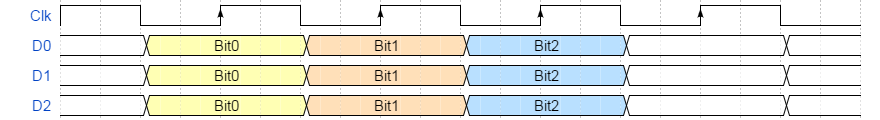(a) Phase Alignment

All SHF DACs are active devices which re-time and re-shape the input signal. This makes the DACs very robust regarding signal impairments and skew at its inputs. Independent from the binary input signal quality one will always receive a perfect PAM signal even if the phase alignment is not as perfect as in the picture above. Nevertheless, the DAC needs to find a valid sampling point and therefore the clock & data input signals must be reasonably good aligned in order to meet the phase margin (hold time) requirements of the device. For example, if an input signal is sampled in its crossing the DAC will produce errors at its outputs.

Proper phase alignment for each single input can be easily verified by operating the DAC in one bit (binary) mode. In case the user has full control of the phase into the DAC (like with a SHF BPG) a perfectly aligned setup can be achieved easily.

(b) Bit Alignment

If above phase alignment requirement is fulfilled the DAC will always produce perfectly shaped PAM signals. For some applications, this is the only requirement one would need to consider.

In case of programming the DAC inputs in order to use it e.g. as an Arbitrary Waveform Generator (AWG) not only the phase alignment has to be considered but also the logical bit alignment. The graphic below shows input D2 is not properly bit aligned and should be shifted by -1 bit.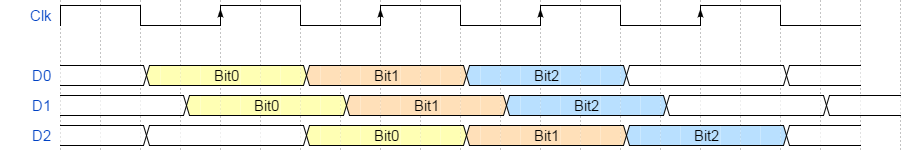In case an error analyzer or a scope is used, there are straight forward possibilities to find out whether the bit streams are bit aligned (please be referred to the SHF literature). In addition, with a SHF 12104 A BPG one has full control of the bit alignment. Shifting D2 by -1 bit would be one click in the software and the DAC would have a perfect bit alignment.

Can a DAC be used to generate PAM-4 with Pre-Emphasis?

Yes, below you will find a simple schematic how this can be done e.g. by utilizing a 4-Bit DAC.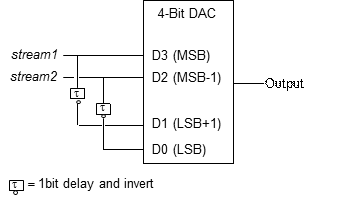In case a SHF BPG with four outputs is available one can set the bit delay and invert the channel just by a few clicks in the software. No additional hardware is required.

In case an SHF BPG with ‘only’ two channels is available the data bar outputs can be used for D0 and D1. Here, external components have to be used for the one bit delay.

Pre-emphases can be a very powerful tool. In the example below a 30 GBaud PAM-4 signal is transmitted through a 2.5 meter SMA cable.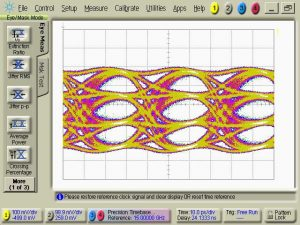Input signal without pre-emphases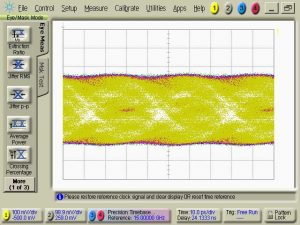Output signal without pre-emphases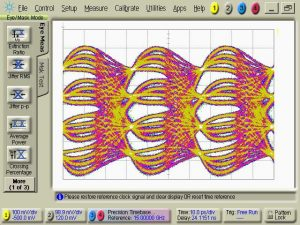Input signal with pre-emphases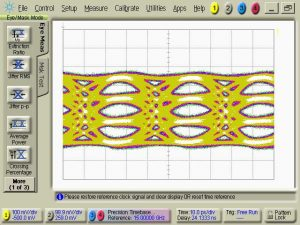Output signal with pre-emphases

What is the difference between passive combining and an active DAC?

It is possible to generate a PAM-4 signal by using a broadband passive combiner. However, this has many drawbacks compared to an active DAC module. Just a few to be mentioned:

Output Power
A DAC usually provides more output signal swing than a passive combiner since the least significant bit into the passive combiner must be attenuated accordingly (usually 6 dB).

Reflection
A passive combiner is transparent in all directions. This means, the signal applied to one input will be present not only at the output but also at the other input. This always has an influence on the output signal quality because multiple reflections impact the overall output signal quality accordingly (due to the imperfect output reflection coefficient (s22) of the driving source). Usually this influence is minimized by inserting attenuators at both pattern generator outputs. This however, further reduces the output voltage.
By using a DAC this problem can be disregarded. In contrary, even if you have a bad signal from your BPG the DAC will always provide a perfectly shaped output as long as it is good enough for sampling by the input latch of the DAC

Skew
The two signals into the passive combiner must be extremely well aligned as even a small skew between the input signals will distort the output signal. In case the used pattern generator does not have an internal fine skew control, an additional external delay line has to be added.  This makes the passive combiner approach rather inconvenient.
Compared to the passive combining the DAC is extremely robust against skew between the driving signals.

Placement at the DUT
A passive combiner must be placed very close to the pattern generator outputs. This means, even if the combiner provides a reasonable good PAM-4 signal it must probably be transmitted through a long cable to the DUT. This will distort the high speed multi-level signal.
The DAC can be placed very close to the DUT. The extension cables can be inserted between the pattern generator’s outputs and DAC. The signal impairments introduced by these cables will not influence the DAC’s outputs as the input DFFs retime and reshape the signal on-chip before the voltage contributions are combined. After all, the DAC is much more robust and can be physically moved on the laboratory bench without any influence on the signal quality.